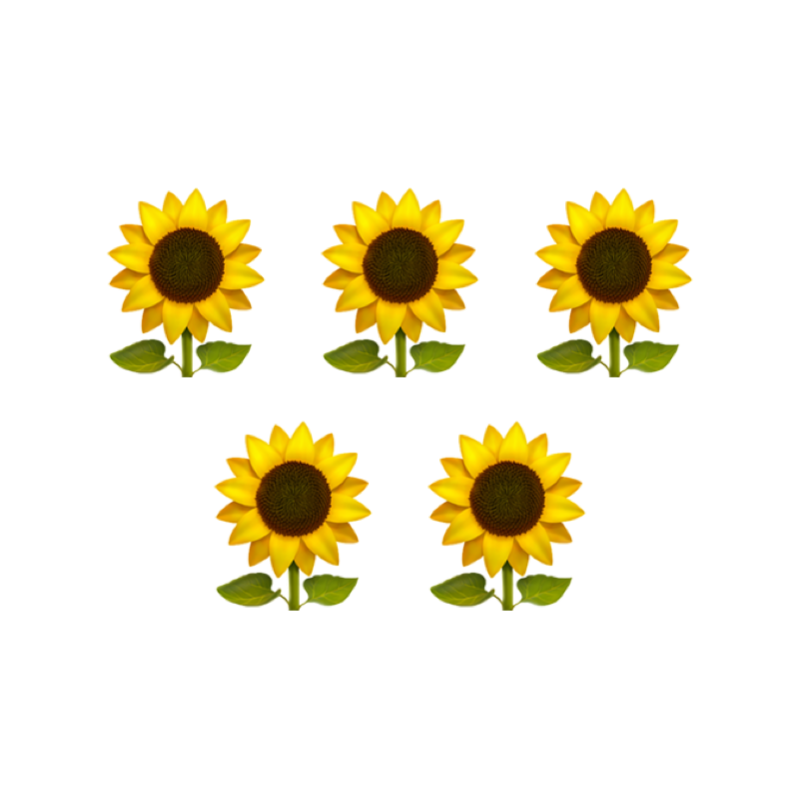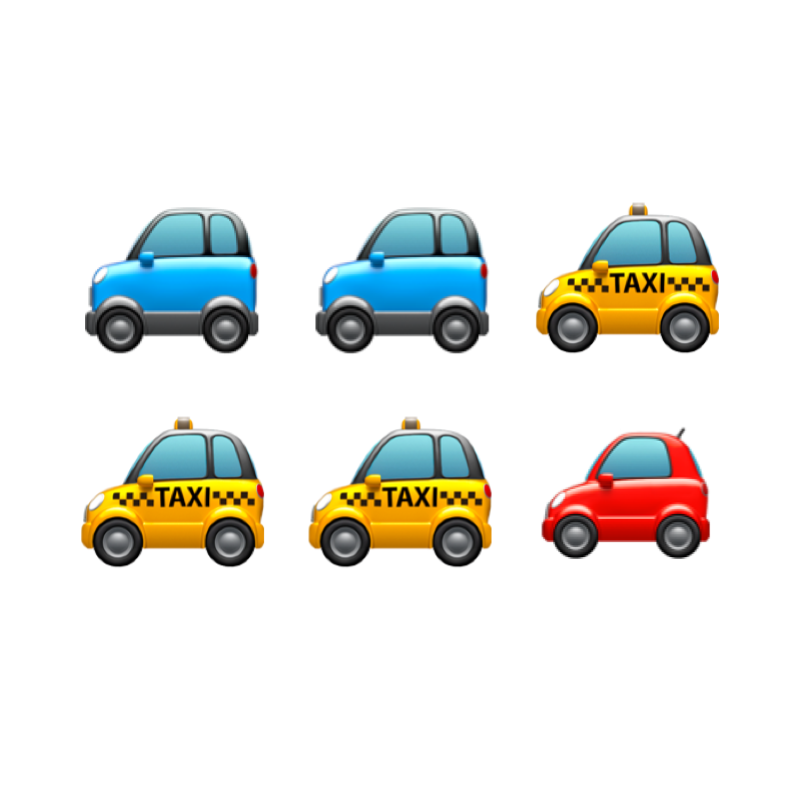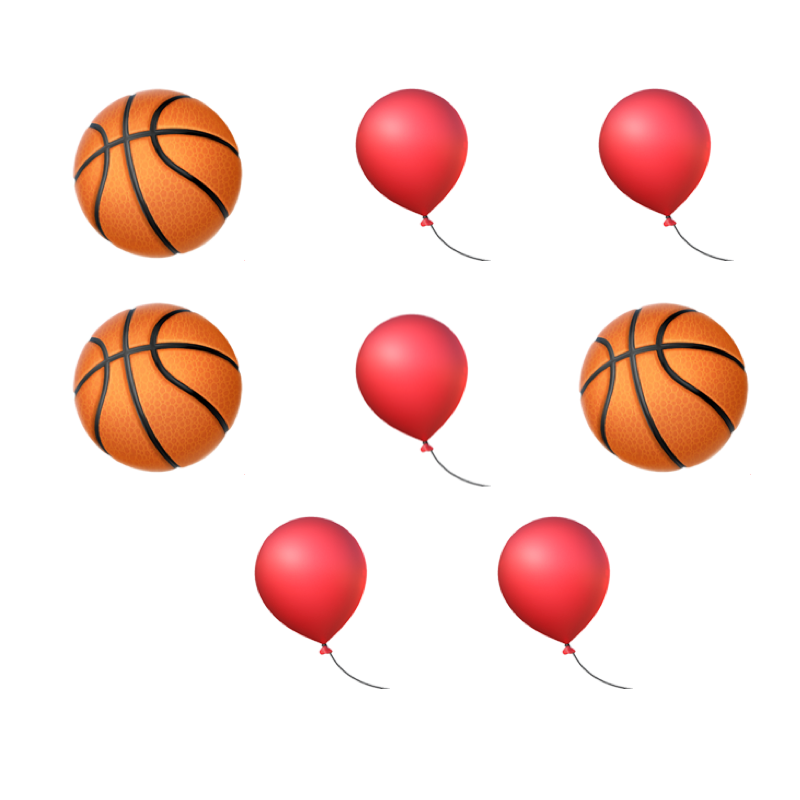maths > fractions

Fractions: Dividing a Group

what you'll learn...

overview

This page explains that fraction can be a part of a whole or can be a part of a group. In the latter case, a fraction represents the number of objects / items out of a total number of objects.
In this, fraction $\frac{p}{q}$$\frac{p}{q}$ represents

$p$$p$ number of items, for every $q$$q$ number of items in the whole group.

one or manyThere are $5$$5$ flowers in the picture.Consider the enclosed picture. The total number of cars is 6. The number of red cars is 1. This is represented as a fraction $\frac{1}{6}$$\frac{1}{6}$. In this, the fraction represents $1$$1$ count out of $6$$6$ total numbers.

The fraction of blue cars in the picture is $\frac{2}{6}$$\frac{2}{6}$. In this, the fraction represents $2$$2$ numbers out of $6$$6$ total numbers.

A group of objects may be divided into sub-groups. The number of objects in the sub-group is represented as a fraction of the whole group.

In the given figure:
The Whole (total number of cars): $6$$6$

Fraction of red cars : $\frac{1}{6}$$\frac{1}{6}$

Fraction of blue cars : $\frac{2}{6}$$\frac{2}{6}$

Fraction of Yellow cabs : $\frac{3}{6}$$\frac{3}{6}$

A fraction is a part of a whole or a part of a group.

Fraction as a part of a group: A fraction represents the number of objects out of a total number of objects.
In this, fraction $\frac{p}{q}$$\frac{p}{q}$ represents
$p$$p$ number of items, for every $q$$q$ number of items in the whole group.

examplesWhat fraction is basketballs in the group?
The answer is '$\frac{3}{8}$$\frac{3}{8}$'.

What fraction is balloons in the group?
The answer is '$\frac{5}{8}$$\frac{5}{8}$'.

summary

»  Fraction of blue cars $\frac{2}{6}$$\frac{2}{6}$→  $6$$6$ cars together make a whole
→  " $2$$2$ cars" is the given number
→  $\frac{1}{6}$$\frac{1}{6}$ is the place value

Outline